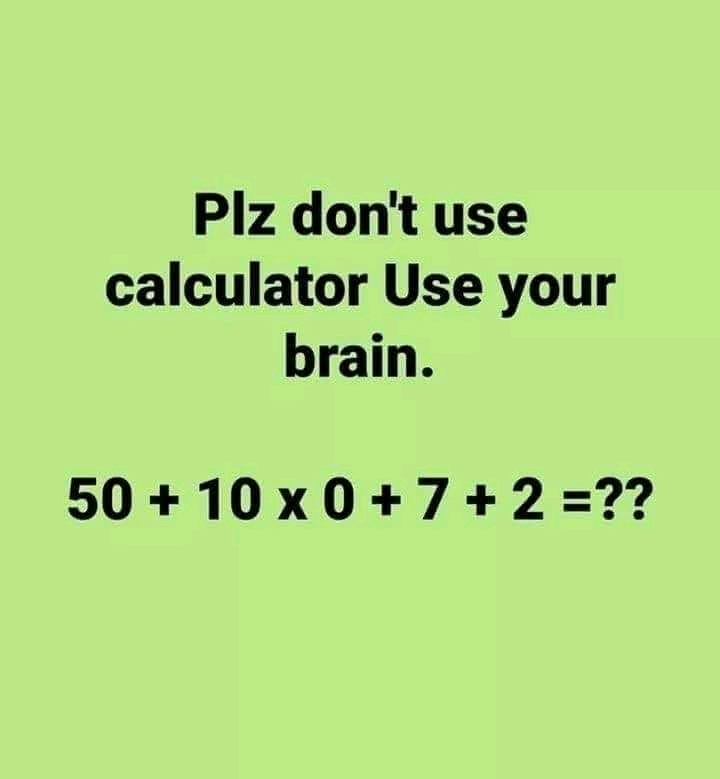0

# What's 50 + 10 × 0 + 7 + 2 = ? Math Quiz answer50 + 10 × 0 + 7 + 2 = ? Math Quiz answer
This 50 + 10 × 0 + 7 + 2 = ? Math quiz is one of those trending on social media today. At a glance, you might be tempted to say "anything Multiply by Zero is equal Zero" from primary school math.

The best way to answer this type of math quiz is to apply BODMAS. I will solve it in detail and give you the correct answer.

Solving math quizzes and puzzles can refresh your mind and keep you relaxed after a long work day.

Be sure to share this " don't use a  calculator" math Brain teaser to your friends and family to tease them.

## Don't use a calculator: What's 50 + 10 × 0 + 7 + 2 = ? Answer

The correct answer would be 59 not zero. This is because anything Multiplying by Zero= zero Math law works if and only if the zero is Multiplying all the expressions.

First I will do Multiplication according to BODMAS.

That's 10 × 0 = 0

The Quiz will now be:

50 + 0 + 7 + 2=?

59 = ?.

Therefore, the correct answer is 59 as mentioned earlier.

Do you have any other view? Share with us.

Also interact with us on Facebook.

Tags